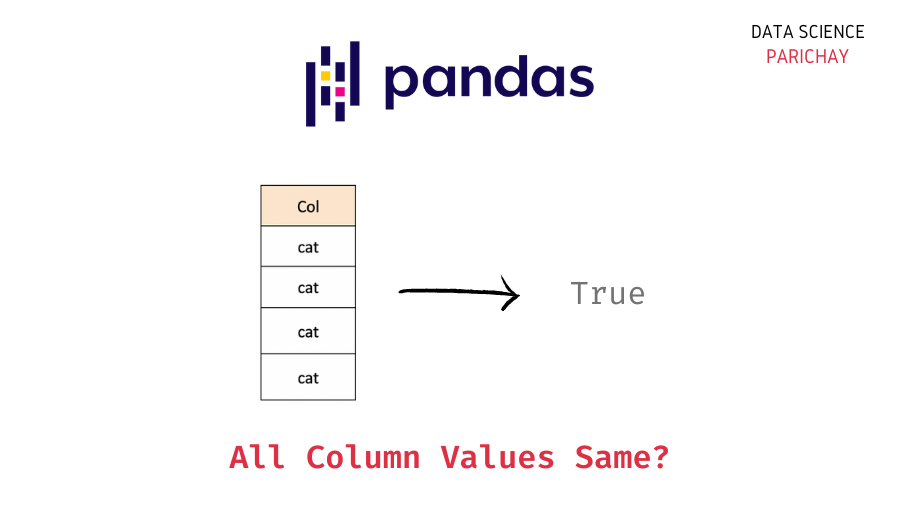# Pandas – Check if a column is all one value

In this tutorial, we’ll try to look at a way to determine if a column in a pandas dataframe is all one value. In simple words, We just need to check if, all the values in a column are the same or not.

## How to check if a column is all one value?

If all of a column’s values are equal to the column’s initial value, we may compare and verify that all of the values in that column are also identical. The following are the steps involved in it:

• Using the DataFrame’s subscript operator, `df['column name']`, choose a column by name. It provides a Pandas Series object representing the column contents.
• Comparing the initial value with the Series object (chosen column). A boolean Series will be returned.
• Verify whether or not each item in the boolean series is True. If the answer is True, then the values in the column are all equal.

Sample Code:

```  import pandas as pd

df = pd.DataFrame({'Col1': ['a', 'a', 'a'], 'Col2': [1, 2, 3]})

Print ((df['Col1'] == df['Col1']).all())```

Output:

`True`

In this method, we first import the pandas and create a dataframe from it. Then we use the above-mentioned steps to check if the column, “Col1” is all one value.

Special Case – Nan Comparision:
In case we have to check whether all the values of the column are Nan or not, the above method is not useful, instead use `.isnull().all()` method.

Sample Code:

`df['Col4'].isnull().all()`

## Examples

Now, Let us look at examples of Each method discussed above to check if all the values in a specific column are the same or not.

📚 Data Science Programs By Skill Level

Introductory

Intermediate ⭐⭐⭐

🔎 Find Data Science Programs 👨‍💻 111,889 already enrolled

Disclaimer: Data Science Parichay is reader supported. When you purchase a course through a link on this site, we may earn a small commission at no additional cost to you. Earned commissions help support this website and its team of writers.

Note: In case, you don’t have a pandas Dataframe, use the below simple method to create one. we use this dataframe for all the examples mentioned below

```import pandas as pd
import numpy as np
df = pd.DataFrame({'Col1': ['a', 'a', 'a'], 'Col2': [1, 2, 3],'Col3':[0,0,0], 'Col4':[np.nan,np.nan,np.nan]})
df```

Output:

### Example – 1

Test case when all the columns values are the same.

Code:

`(df['Col1'] == df['Col1']).all()`

Output:

`True`

### Example – 2

Test case when all the columns values are not the same.

Code:

`(df['Col2'] == df['Col2']).all()`

Output:

`False`

### Example – 3

Test case when all the columns values are zero.

Code:

`(df['Col3'] == 0).all()`

Output:

`True`

### Example – 4

Test case when all the columns values are Nan.

Code:

`df['Col4'].isnull().all()`

Output:

`True`

## Summary

In this tutorial, we looked at a way to determine if a column is all one value. In simple words, we just need to check if all the values in a column are the same or not.
It is done simply through the following steps:

• Selecting the required column of the dataframe using the subscript operator.
• Take the first value of the column and then compare it with all the other values in the column.
• Verify whether or not each item in the boolean series is True.
• If the values are true, then all values in the column are the same.

You might also be interested in –

•